# Constructive logic

(diff) ← Older revision | Latest revision (diff) | Newer revision → (diff)

A branch of mathematical logic studying arguments concerning constructive objects (cf. Constructive object) and constructions. In this sense constructive logic is broader than the logic of constructive mathematics. The most prominent difference from traditional (classical) logic consists in the absence of the law of the excluded middle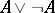and the law of double negation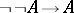(cf. Double negation, law of).

When describing pure logical systems (propositional and predicate calculus), the terms "constructive" , "intuitionistic" , "Heyting" are often considered to be synonymous (see Heyting formal system). Constructive arithmetic sometimes refers to Heyting arithmetic and sometimes to its extension obtained by adding Markov's principle (see Constructive selection principle) and the scheme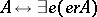, expressing the equivalence of a formula and the assertion of its realizability (see Constructive semantics). This extended system, which is sufficient for the proof of the fundamental results of constructive mathematical analysis, is not, in contrast to the Heyting one, a subsystem of classical arithmetic: The law of the excluded middle,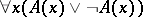, is refutable in it. Systems of intuitionistic logic, including means of describing specific intuitionistic concepts, are sometimes also taken to belong to constructive logic. The general manner in which most of the systems of constructive logic reflect the specific constructive understanding of the disjunction sign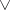and the existential quantifier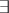is by giving an explicit realization of these connectives. The derivability of(or of) implies that of one of the formulas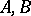(or of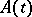for some term). In the case of applied systems (arithmetic, analysis) it is required that the formulas under consideration be closed. The majority of systems of constructive logic (including all Heyting systems) are sound with respect to the various notions of realizability, including the Kleene realizability and Gödel interpretation: All derivable formulas are realizable, and as a consequence they are true in constructive semantics. On the other hand, formal systems of constructive logic are usually incomplete with respect to natural constructive semantics. For systems containing arithmetic this follows from the Gödel incompleteness theorem.

The set of realizable predicate formulas is not recursively enumerable, therefore constructive predicate calculus is incomplete with respect to realizability, while its completeness with respect to "naive" constructive semantics would imply the intuitionistic truth of the constructive selection principle. Constructive propositional calculus is also incomplete with respect to realizability, but is complete under a certain interpretation in terms of Post systems. The arithmetic semi-formal system, which is complete with respect to the constructive semantics of Markov–Shanin, is obtained by adding to formal constructive arithmetic with the schemeand the constructive selection principle, the effective-rule: From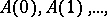one can infer. For Heyting systems, completeness theorems have been established with respect to the model-theoretic semantics of Kripke and Beth which use "possible worlds" (these semantics are related to set-theoretic forcing), and also with respect to algebraic and topological models.

Classical formal systems usually can be embedded in the corresponding systems of constructive logic (with preservation of derivability from hypotheses) by means of the Gödel double negation (translation), defined by putting the double negation signin front of all subformulas. Therefore systems of arithmetic, analysis and the theory of types (cf. Types, theory of) based on classical logic can be isomorphically embedded in the corresponding systems based on constructive logic. There are systems of set theory based on constructive logic in which classical systems can be embedded. The Heyting systems are embedded in modal extensions of the classical ones by means of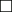-translation, that is, by putting the necessity signin front of all subformulas. Herecan be read as "provable" , or, better, as "provable and true" .

In certain systems of constructive logic, statements that are false in the classical interpretation, for example, the negation of the law of the excluded middle, or specific intuitionistic statements concerning choice sequences, are valid. Such systemsreduce to classical systems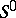by means of a suitable notion of realizability. One has proved that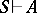implies the existence of asuch that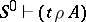. Moreover, ifis numerical equality, then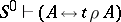. This implies the consistency ofrelative to.

Constructive logic also studies the validity of statements in non-traditional languages that differ from the languages of predicate logic, arithmetic, analysis, etc. Along with the traditional negation, the strong negationis studied, which provides the construction of a counterexample. Formany of the laws of classical logic are valid, for example,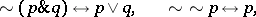but the theorem on substitution of equivalents is true only in the form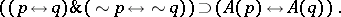Close to the system of strong negation are systems based on a symmetric treatment of truth and falsehood. The semantics for these specify not only the form of the constructions justifying truth but also the form of the constructions justifying falsity of the statements under consideration.

The negation-free Griss–Nelson logic seeks to avoid the use of negation and tends to consider only non-empty properties. The language of such a logic contains the connective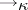, where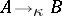means roughly that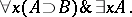In the theory of constructions one studies the very rules of construction and proof that lie at the foundation of constructive mathematics. Constructions are built from primitive ones by means of a fixed collection of combinators and the operation of applying a function to an argument. Formulas are built from equalities by means of the connectives of propositional logic and the provability predicate, whereis to be read as "a is a proof of the fact that ax is true for all x" . All classical tautologies (including the law of the excluded middle) are taken to be axioms, that is, the relation of "being a proof" is assumed to be decidable. There is a sound and faithful (complete) interpretation of the Heyting systems in the theory of constructions.

Quantifier-free systems are used in constructive logic to obtain finitistic proofs (in some sense or other) of statements or majorants of them (see Constructive semantics). Many traditional systemsof constructive logic can be embedded in quantifier-free systems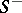in such a way that the derivability inof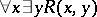, with a quantifier-free formula, implies derivability inof the formulafor suitable. Ifis the first-order arithmetic without induction, thenis a primitive-recursive arithmetic; ifis a Heyting arithmetic, thenis a system of Gödel primitive-recursive functionals.

For formal systems of constructive logic normalization theorems have been proved: Any derivation can, by a finite number of standard transformations (reductions), be reduced to a normal form containing no "superfluous" parts (see Gentzen formal system). Normal derivations possess (fully, or in the case arithmetic or stronger systems, partially), the subformula property.

There is a connection between constructive logic and category theory. E.g., the notion of a "Cartesian-closed categoryCartesian-closed category" corresponds to the Gentzen propositional calculus.

Sometimes constructive logic is understood to include all logical arguments in which it is required that all objects under study be constructive, independently of the logic used.

How to Cite This Entry:
Constructive logic. Encyclopedia of Mathematics. URL: http://encyclopediaofmath.org/index.php?title=Constructive_logic&oldid=13038
This article was adapted from an original article by G.E. Mints (originator), which appeared in Encyclopedia of Mathematics - ISBN 1402006098. See original article